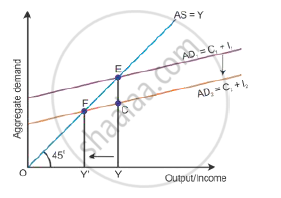# Explain the Concept of 'Deficient Demand' in Macroeconomics. - Economics

Explain the concept of 'deficient demand' in macroeconomics.

What is 'deficient demand'?

#### Solution

Deficient demand means a situation when the aggregate demand is short of the aggregate supply corresponding to full employment in the economy. It leads to a fall in the general price level and results in deflation, i.e. AD < AS. Aggregate demand is shown by the AD curve and aggregate supply is shown by the AS curve (as shown in the diagram below). While the aggregate demand curve and the aggregate supply curve intersect each other, the full employment equilibrium is attained at Point E. OY is the full employment level of output, and EY is the aggregate demand at full employment level of output. If the aggregate demand decreases below the full employment level of output from EY to CY, then the economy will have deficient demand, (EY − CY = EC).Concept: Concept of Aggregate Demand and Aggregate Supply
Is there an error in this question or solution?